Topic
PDFs
Data & Scripts

Exercises

Introduction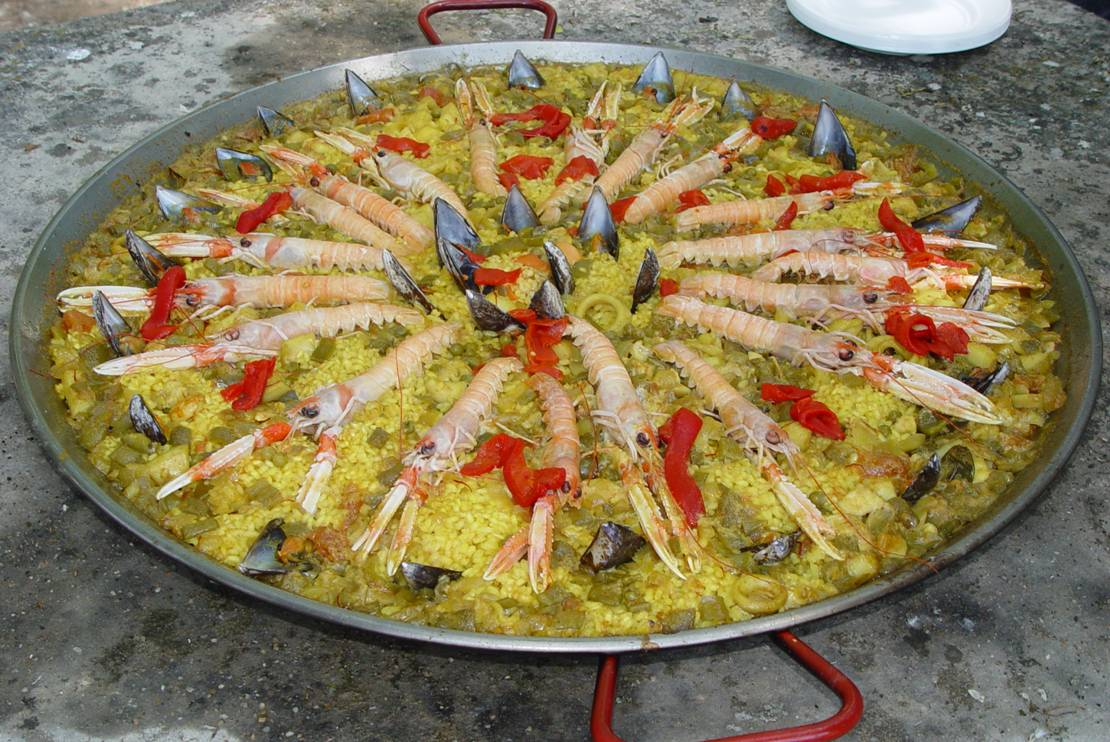http://cran.r-project.org/doc/manuals/R-intro.pdfInstructions for exercises

Introduction to Probability

Random variables and Probability distributions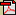Exercise 1b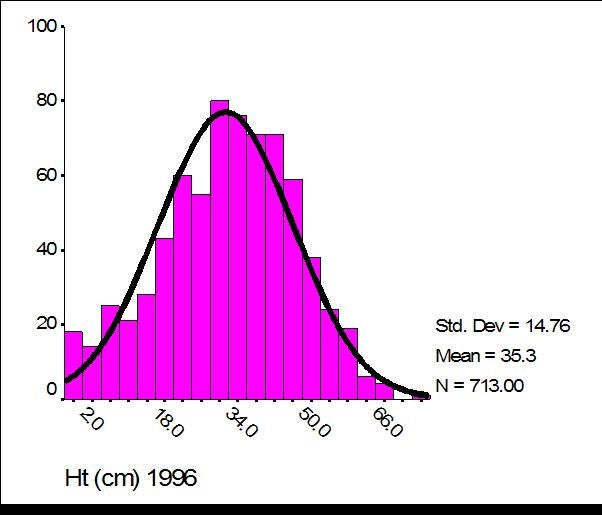Managing and curating dataExercise 2Experimental designSummary Statistics and samplingHypericum cumulicola population levelExercise 3Statistical modelsConfidence intervalsExercise 4

#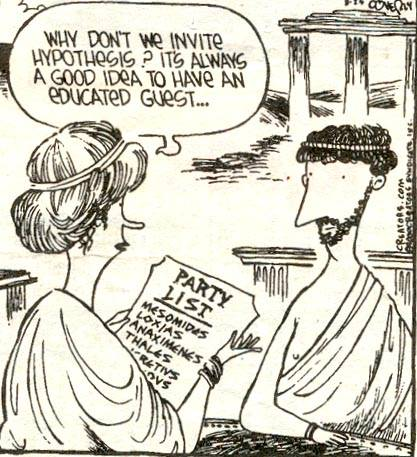Three Frameworks for Statistical AnalysisSimple linear RegressionTransformationsUseful properties of the normal distribution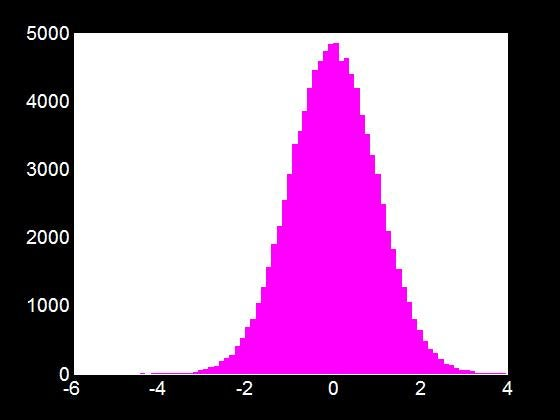Exam 1

Return Date:

October 9 @ 3:00 PMMultiple RegressionModel selectionExercise 8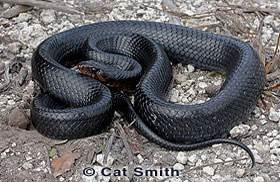Bibliography model selection
General linear models (ANOVA)Nested and Block designsFactorial designs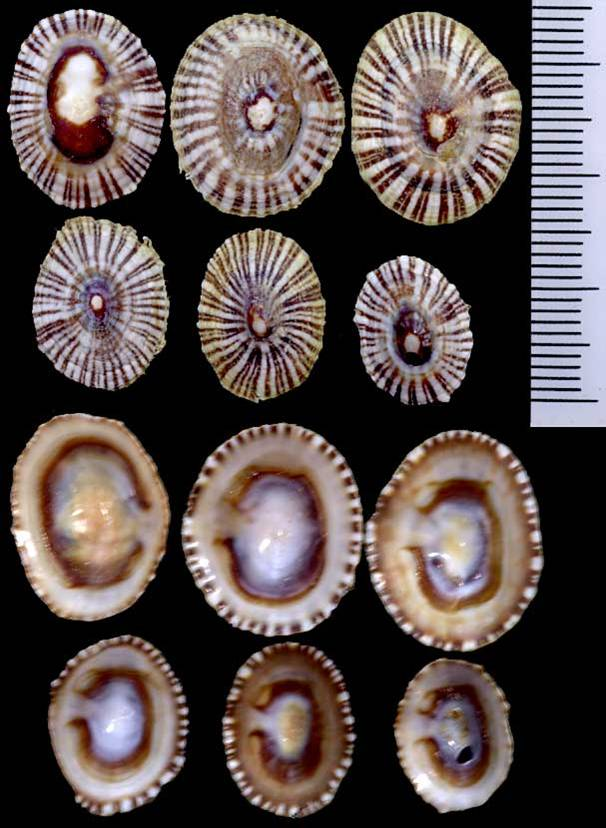General linear models (ANCOVA)Regression of counts

(Non-normal errors)Snakes scriptSnakes dataMultiple ComparisonsMultiple comparisons – R demo (11)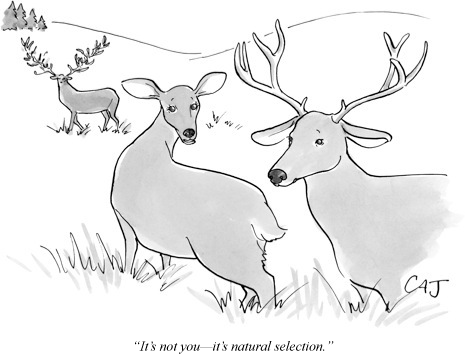Logistic RegressionLogistic Regression – R demo (12)Analysis of Categorical DataFinal ExamFinal Exam

Return Date:

December 6 @ 11:59 PM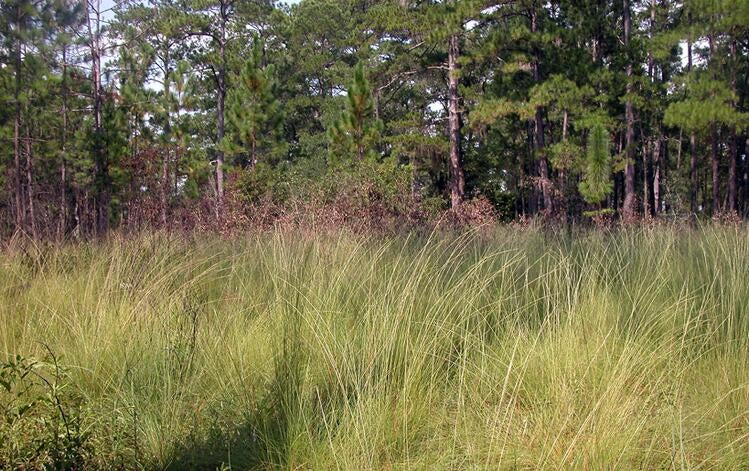Thanks for the opportunity of sharing ideas with you!